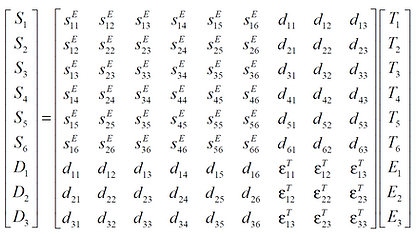top of page# Fundamental Equations of PiezoelectricityThe fundamental equations of the piezoelectricity are derived from the thermodynamic energy balance. The energies involved are mechanical, electrical and thermal. In order to make it not over-complicated we don’t look at the thermal energy at this point. For our purpose we need the interaction between the electrical and mechanical behaviour of the material.

In order to get this right we need to dig a little further.

The electrical behaviour of a dielectric (or unstressed piezo material ) under the influence of an electric field is described by the field strength E  and the dielectric displacement D.

D = ε E

ε  is the permittivity of the material.

The displacement D is also called electric flux density or charge density.

The mechanical behaviour of the same material at zero electric field strength is defined by two mechanical quantities, the applied stress T and the strain S :

S = s T

is the compliance of the material.

For a piezoelectric material we need to include the polarization. The full description of mechanical-electrical interaction can be made to a good approximation by linear relations as follows:

In both these relations the piezoelectric charge constant d appears.  We conclude that d can be defined as a quotient of either S and E or D and T.

Actually the above set of equations are tensor equations with 3 electrical quantities (in the 1, 2 and 3 directions) and 6 mechanical quantities namely the normal stress in three directions and the shear stress about the three axes. This means that of each piezoelectric parameter in the most general case up to 18 variables can exist, indicated by subscripts.

If we write it out (for the fun) it looks like this:

[S] = m/m         (meter per meter)

[D] = C/m²        (Coulombs per meter squared)So for example D1 would be calculated asLuckily in most cases many elements of the above matrix are zero or due to the symmetry of the crystal at least equal to another element.

The choice of independent variables (mechanical T and electrical E ) is arbitrary.

If we choose the displacement D instead of E as independent electrical variable we get the following pair of relations:Now the piezoelectric voltage constant g appears and we see that g can be expressed as a quotient of  E and T or S and D.

A given pair of piezoelectric equations corresponds to a particular choice of independent variables. In a similar way it is possible to arrive at yet different pairs of relations:Terms c and c are the elastic stiffnesses (stress per unit strain) and h and e are piezoelectric constants. They are seldom used in practice and mentioned here only for the sake of completeness.

bottom of page